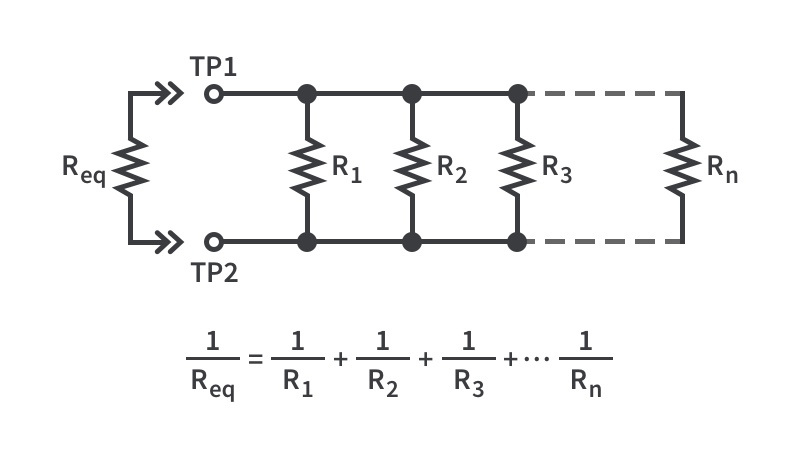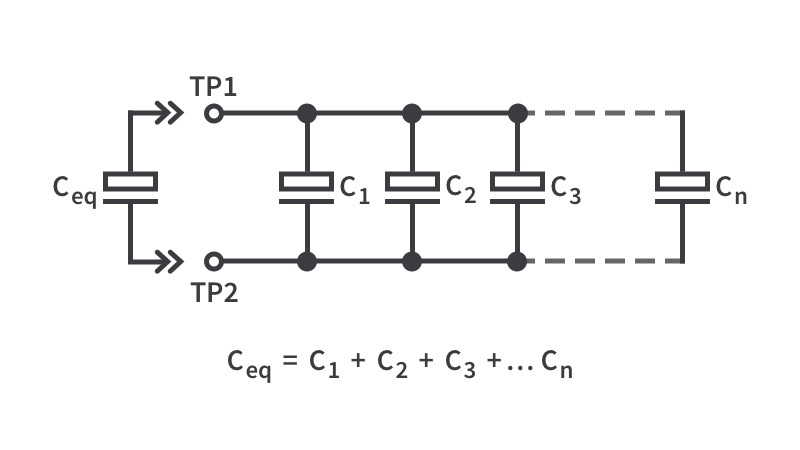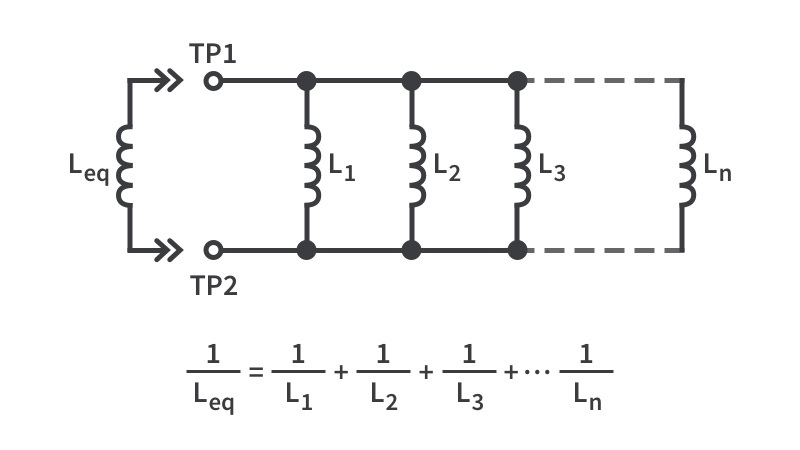# Parallel EquivalenceInputOutputCalculating the parallel equivalence of a resistor, especially when there are more than two of them, is certainly more complicated than finding the series equivalence. However, you can use the formula above to do it yourself and then check to make sure you did it correctly with this convenient calculator!InputOutputCalculating the parallel equivalence of a capacitor is quite straightforward as you simply sum the capacitance of all the capacitors together. Double-checking your work is always good, so we’ve provided this “Parallel Equivalence for Capacitors” calculator to make your life even better.InputOutput